# How to Insert a Calculated Item into Excel Pivot Table!

In this article, I’ll show you how you can insert a calculated item into an already created Excel Pivot Table. Some Excel users avoid these two items (calculated field and calculated item) due to the complexity associated with these two features.

Related: How to Create an Average Calculated Field in Excel Pivot Table

Let’s make you understand how calculated item works with Excel pivot table. In the image below, you’re seeing an example data. We shall this data table to create an Excel Pivot Table and then we shall insert a calculated item into that pivot table.

So, let’s start.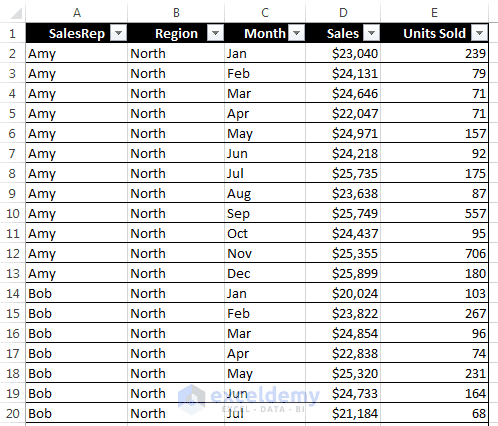This figure shows part of a table. We shall use this table to work with calculated fields and calculated items.

The above table has five columns and 48 rows. The rows of the table contain the records of monthly sales by a particular sales representative. For example, Amy is a sales representative for the North region, she has sold 239 units in the month of February and her total sales were \$23,040.

The following figure shows a pivot table created from the table. To create this pivot table, we have placed the Month field in the Rows area, SalesRep field in the Columns area and Sales field in the Values area.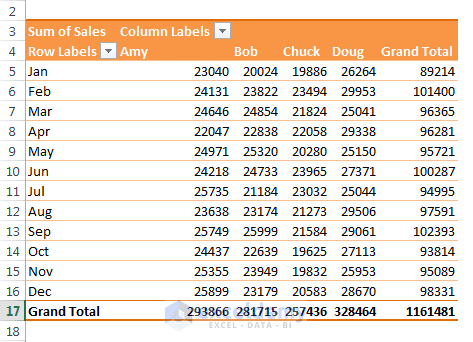This pivot table is created from the above table. We have placed Month, SalesRep in Rows and Columns area, and Sales in Values area.

calculated-fields-and-items.xlsx

## Creating a calculated item in a pivot table

In this example, we are going to create four calculated items that will be inserted into our previously created pivot table. Each item will calculate commission earnings of a sales representative in a certain quarter.

The quarterly commission will be calculated in the following schedule:

• Quarter 1:10% of January, February, and March sales
• Quarter 2:11% of April, May, and June sales
• Quarter 3:12% of July, August, and September sales
• Quarter 4:12.5% of October, November, and December sales

Note: If you want to modify the source data to get quarterly commission of sales representatives, you have to insert 16 new rows. Each row will contain quarterly commission of a sales representative. So, in our example, creating four calculated items in the pivot table is an easier task.

Read More: Excel Pivot Table Terminology

We have used the following process to create a calculated item in our pivot table:

### Step 1

Select any label in Row Labels or Column Labels area of the pivot table and choose PivotTable Tools ➪ Analyze ➪ Calculations ➪ Fields, Items & Sets ➪ Calculated Item. The Insert Calculated Item dialog box will appear.

### Step 2

Enter a descriptive name for the new item in the Name field and enter the formula in the Formula field in Insert Calculated Item as shown in the following figure. You can use items in other fields in the formula but you can’t use worksheet functions. In our example, we have entered Qtr1 Commission in the Name field and this formula in the formula field: =10%*(Jan+Feb+Mar).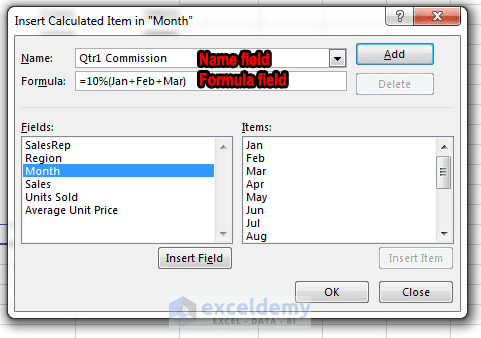Insert Calculated Item dialog box. Enter an item name in the name field and enter a relevant formula in the formula field.

### Step 3

Click Add button, the item Qtr1 Commission is inserted into the Items list.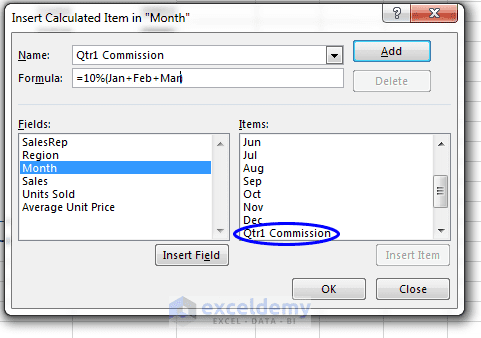Qtr1 Commission item is inserted into Items list.

### Step 4

Repeat Steps 2 and 3 to create three more calculated items: Name field: Qtr2 Commission, Formula field: =11%*(Apr+May+Jun); Name field: Qtr3 Commission, Formula field: = 12%*(Jul+Aug+Sep); Name field: Qtr4 Commission, Formula field: = 12.5%*(Oct+Nov+Dec).

### Step 5

Click OK to close the dialog box.

Note: A calculated item does not appear in the PivotTable Fields task pane. Only calculated fields are listed in the fields list in the task pane.

Important Caution: If you use a calculated item in your pivot table, you have to turn off the Grand Total display for columns to avoid double counting. In this example, the Grand Total also calculates the calculated items, so the commission amounts are added to the sales amounts. To turn off Grand Totals option, you have to choose PivotTable Tools ➪ Design ➪ Layout ➪ Grand Totals ➪ On for Rows Only.

Read More: Creating an Excel Pivot Table Manually

When you have finished creating four calculated items, these items will appear in the pivot table. The following figure shows the pivot table after adding the four calculated items.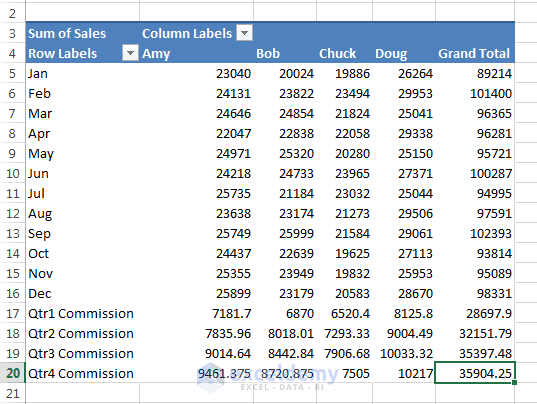Pivot table after we have added four calculated items.

Observe that the calculated items are added to the end of the Month items. You can rearrange the items. To rearrange, select the cell, drag its border, and place it to your desired location. You can also create two groups: one for sales numbers and another one for commission earnings. The following figure shows the pivot table where we have created two groups, adding subtotals and using some style.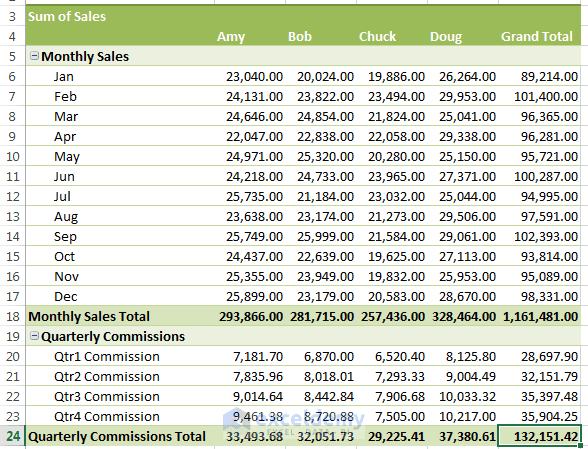Pivot Table after making two groups and using style to take a professional look

Happy Excelling 🙂

#### Kawser

Hello! Welcome to my Excel blog! It took me some time to be a fan of Excel. But now I am a die-hard fan of MS Excel. I learn new ways of doing things with Excel and share here. Not only how to guide on Excel, but you will get also topics on Finance, Statistics, Data Analysis, and BI. Stay tuned!

1 Comment